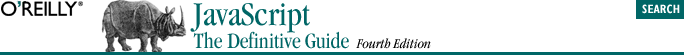home | O'Reilly's CD bookshelfs | FreeBSD | Linux | Cisco | Cisco Exam## 5.2. Operator Overview

If you are a C, C++, or Java programmer, most of the JavaScript operators should already be familiar to you. Table 5-1 summarizes the operators; you can refer to this table for reference. Note that most operators are represented by punctuation characters such as + and =. Some, however, are represented by keywords such as delete and instanceof. Keyword operators are regular operators, just like those expressed with punctuation; they are simply expressed using a more readable and less succinct syntax.

#### Table 5-1. JavaScript operators

P

A

Operator

Operand type(s)

Operation performed

15

L

.

object, identifier

Property access

L

[]

array, integer

Array index

L

( )

function, arguments

Function call

R

new

constructor call

Create new object

14

R

++

lvalue

Pre- or post-increment (unary)

R

--

lvalue

Pre- or post-decrement (unary)

R

-

number

Unary minus (negation)

R

+

number

Unary plus (no-op)

R

~

integer

Bitwise complement (unary)

R

!

boolean

Logical complement (unary)

R

delete

lvalue

Undefine a property (unary)

R

typeof

any

Return data type (unary)

R

void

any

Return undefined value (unary)

13

L

*, /, %

numbers

Multiplication, division, remainder

12

L

+, -

numbers

L

+

strings

String concatenation

11

L

<<

integers

Left shift

L

>>

integers

Right shift with sign-extension

L

>>>

integers

Right shift with zero extension

10

L

<, <=

numbers or strings

Less than, less than or equal

L

>, >=

numbers or strings

Greater than, greater than or equal

L

instanceof

object, constructor

Check object type

L

in

string, object

Check whether property exists

9

L

==

any

Test for equality

L

!=

any

Test for inequality

L

===

any

Test for identity

L

!==

any

Test for non-identity

8

L

&

integers

Bitwise AND

7

L

^

integers

Bitwise XOR

6

L

|

integers

Bitwise OR

5

L

&&

booleans

Logical AND

4

L

||

booleans

Logical OR

3

R

?:

boolean, any, any

Conditional operator (3 operands)

2

R

=

lvalue, any

Assignment

R

*=, /=, %=, +=, -=, <<=, >>=, >>>=, &=, ^=, |=

lvalue, any

Assignment with operation

1

L

,

any

Multiple evaluation

### 5.2.3. Operator Precedence

In Table 5-1, the column labeled "P" specifies the precedence of each operator. Operator precedence controls the order in which operations are performed. Operators with higher numbers in the "P" column are performed before those with lower numbers.

Consider the following expression:

`w = x + y*z; `

Operator precedence can be overridden with the explicit use of parentheses. To force the addition in the previous example to be performed first, we would write:

`w = (x + y)*z; `